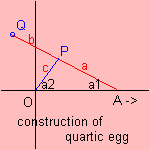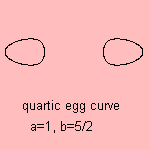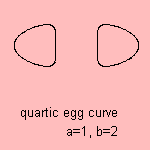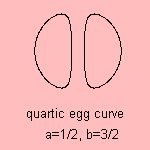# quartic egg curve

## quartic

last updated: 2004-05-09The curve is formed by a set of two attached sticks, as follows:
A stick OP is fixed in O, and attached to a second stick in point P. The second stick AQ is free to move (in point A) along the x-axis.
Then the path of Q forms the quartic egg curve.

An Italian animation shows the construction very clearly.

The equation above follows from the expressions for the x- and y-coordinate of the point Q 1).notes

1) As follows (let c=1):
xQ = b cos a1 - cos a2
yQ = b sin a1 + sin a2
with
sin a2 = a sin a1

Remark: the parameters a and b have been transposed.